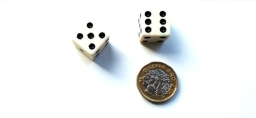# Probability 80785

We roll the dice and then toss the coin as many times as the number that came up on the dice. What is the probability that the coin lands head at least once?

p =  0.8359

### Step-by-step explanation:Did you find an error or inaccuracy? Feel free to write us. Thank you!

Tips for related online calculators
Need help calculating sum, simplifying, or multiplying fractions? Try our fraction calculator.
Would you like to compute the count of combinations?